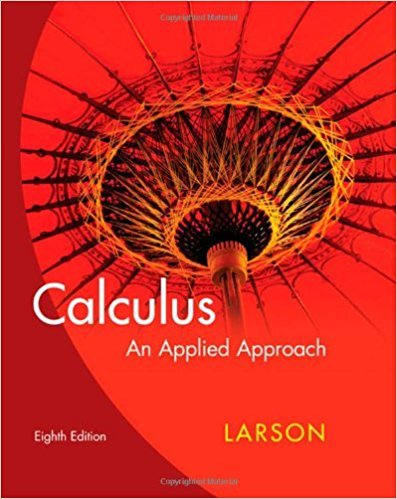×
×

# Solutions for Chapter 10.1: Sequences## Full solutions for Calculus: An Applied Approach | 8th Edition

ISBN: 9780618958252Solutions for Chapter 10.1: Sequences

Solutions for Chapter 10.1
4 5 0 277 Reviews
20
4
##### ISBN: 9780618958252

This expansive textbook survival guide covers the following chapters and their solutions. Calculus: An Applied Approach was written by and is associated to the ISBN: 9780618958252. This textbook survival guide was created for the textbook: Calculus: An Applied Approach , edition: 8. Since 80 problems in chapter 10.1: Sequences have been answered, more than 21935 students have viewed full step-by-step solutions from this chapter. Chapter 10.1: Sequences includes 80 full step-by-step solutions.

Key Calculus Terms and definitions covered in this textbook
• Absolute value of a vector

See Magnitude of a vector.

• Angular speed

Speed of rotation, typically measured in radians or revolutions per unit time

• Arithmetic sequence

A sequence {an} in which an = an-1 + d for every integer n ? 2 . The number d is the common difference.

• Coefficient matrix

A matrix whose elements are the coefficients in a system of linear equations

• Descriptive statistics

The gathering and processing of numerical information

• Expanded form

The right side of u(v + w) = uv + uw.

• Expanded form of a series

A series written explicitly as a sum of terms (not in summation notation).

• Future value of an annuity

The net amount of money returned from an annuity.

• Law of sines

sin A a = sin B b = sin C c

See Polynomial function in x

• Linear function

A function that can be written in the form ƒ(x) = mx + b, where and b are real numbers

• Natural logarithm

A logarithm with base e.

• Parameter

See Parametric equations.

• Pascal’s triangle

A number pattern in which row n (beginning with n = 02) consists of the coefficients of the expanded form of (a+b)n.

• Piecewise-defined function

A function whose domain is divided into several parts with a different function rule applied to each part, p. 104.

• Product of a scalar and a vector

The product of scalar k and vector u = 8u1, u29 1or u = 8u1, u2, u392 is k.u = 8ku1, ku291or k # u = 8ku1, ku2, ku392,

• Triangular number

A number that is a sum of the arithmetic series 1 + 2 + 3 + ... + n for some natural number n.

• Vertical line test

A test for determining whether a graph is a function.

• Zero vector

The vector <0,0> or <0,0,0>.

×